Open in App
Not now

# Selecting rows in pandas DataFrame based on conditions

• Difficulty Level : Medium
• Last Updated : 10 Jun, 2021

Let’s see how to Select rows based on some conditions in Pandas DataFrame.

### Selecting rows based on particular column value using `'>', '=', '=', '<=', '!=' `operator.

Code #1 : Selecting all the rows from the given dataframe in which ‘Percentage’ is greater than 80 using basic method.

 `# importing pandas ` `import` `pandas as pd ` ` `  `record ``=` `{ ` ` `  ` ``'Name'``: [``'Ankit'``, ``'Amit'``, ``'Aishwarya'``, ``'Priyanka'``, ``'Priya'``, ``'Shaurya'` `], ` ` ``'Age'``: [``21``, ``19``, ``20``, ``18``, ``17``, ``21``], ` ` ``'Stream'``: [``'Math'``, ``'Commerce'``, ``'Science'``, ``'Math'``, ``'Math'``, ``'Science'``], ` ` ``'Percentage'``: [``88``, ``92``, ``95``, ``70``, ``65``, ``78``] } ` ` `  `# create a dataframe ` `dataframe ``=` `pd.DataFrame(record, columns ``=` `[``'Name'``, ``'Age'``, ``'Stream'``, ``'Percentage'``]) ` ` `  `print``(``"Given Dataframe :\n"``, dataframe)  ` ` `  `# selecting rows based on condition ` `rslt_df ``=` `dataframe[dataframe[``'Percentage'``] > ``80``] ` ` `  `print``(``'\nResult dataframe :\n'``, rslt_df) `

Output :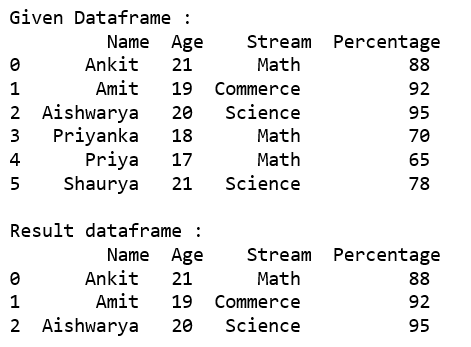Code #2 : Selecting all the rows from the given dataframe in which ‘Percentage’ is greater than 80 using `loc[]`.

 `# importing pandas ` `import` `pandas as pd ` ` `  `record ``=` `{ ` `  ``'Name'``: [``'Ankit'``, ``'Amit'``, ``'Aishwarya'``, ``'Priyanka'``, ``'Priya'``, ``'Shaurya'` `], ` `  ``'Age'``: [``21``, ``19``, ``20``, ``18``, ``17``, ``21``], ` `  ``'Stream'``: [``'Math'``, ``'Commerce'``, ``'Science'``, ``'Math'``, ``'Math'``, ``'Science'``], ` `  ``'Percentage'``: [``88``, ``92``, ``95``, ``70``, ``65``, ``78``]} ` ` `  `# create a dataframe ` `dataframe ``=` `pd.DataFrame(record, columns ``=` `[``'Name'``, ``'Age'``, ``'Stream'``, ``'Percentage'``]) ` ` `  `print``(``"Given Dataframe :\n"``, dataframe)  ` ` `  `# selecting rows based on condition ` `rslt_df ``=` `dataframe.loc[dataframe[``'Percentage'``] > ``80``] ` ` `  `print``(``'\nResult dataframe :\n'``, rslt_df) `

Output :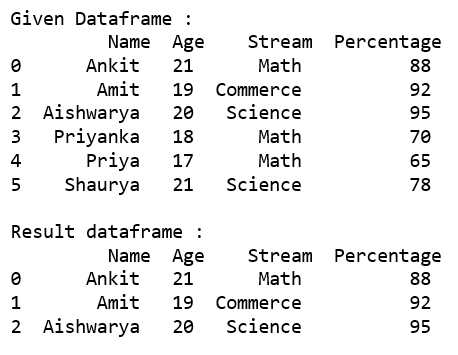Code #3 : Selecting all the rows from the given dataframe in which ‘Percentage’ is not equal to 95 using `loc[]`.

 `# importing pandas ` `import` `pandas as pd ` ` `  `record ``=` `{ ` `  ``'Name'``: [``'Ankit'``, ``'Amit'``, ``'Aishwarya'``, ``'Priyanka'``, ``'Priya'``, ``'Shaurya'` `], ` `  ``'Age'``: [``21``, ``19``, ``20``, ``18``, ``17``, ``21``], ` `  ``'Stream'``: [``'Math'``, ``'Commerce'``, ``'Science'``, ``'Math'``, ``'Math'``, ``'Science'``], ` `  ``'Percentage'``: [``88``, ``92``, ``95``, ``70``, ``65``, ``78``]} ` ` `  `# create a dataframe ` `dataframe ``=` `pd.DataFrame(record, columns ``=` `[``'Name'``, ``'Age'``, ``'Stream'``, ``'Percentage'``]) ` ` `  `print``(``"Given Dataframe :\n"``, dataframe)  ` ` `  `# selecting rows based on condition ` `rslt_df ``=` `dataframe.loc[dataframe[``'Percentage'``] !``=` `95``] ` ` `  `print``(``'\nResult dataframe :\n'``, rslt_df) `

Output :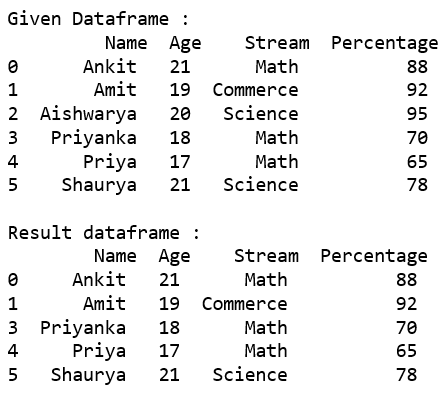### Selecting those rows whose column value is present in the list using `isin()` method of the dataframe.

Code #1 : Selecting all the rows from the given dataframe in which ‘Stream’ is present in the options list using basic method.

 `# importing pandas ` `import` `pandas as pd ` ` `  `record ``=` `{ ` `  ``'Name'``: [``'Ankit'``, ``'Amit'``, ``'Aishwarya'``, ``'Priyanka'``, ``'Priya'``, ``'Shaurya'` `], ` `  ``'Age'``: [``21``, ``19``, ``20``, ``18``, ``17``, ``21``], ` `  ``'Stream'``: [``'Math'``, ``'Commerce'``, ``'Science'``, ``'Math'``, ``'Math'``, ``'Science'``], ` `  ``'Percentage'``: [``88``, ``92``, ``95``, ``70``, ``65``, ``78``]} ` ` `  `# create a dataframe ` `dataframe ``=` `pd.DataFrame(record, columns ``=` `[``'Name'``, ``'Age'``, ``'Stream'``, ``'Percentage'``]) ` ` `  `print``(``"Given Dataframe :\n"``, dataframe)  ` ` `  `options ``=` `[``'Math'``, ``'Commerce'``] ` ` `  `# selecting rows based on condition ` `rslt_df ``=` `dataframe[dataframe[``'Stream'``].isin(options)] ` ` `  `print``(``'\nResult dataframe :\n'``, rslt_df) `

Output :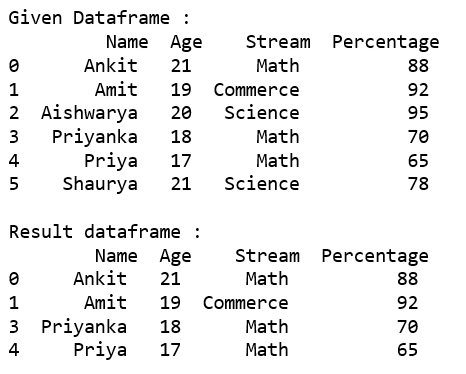Code #2 : Selecting all the rows from the given dataframe in which ‘Stream’ is present in the options list using `loc[]`.

 `# importing pandas ` `import` `pandas as pd ` ` `  `record ``=` `{ ` `  ``'Name'``: [``'Ankit'``, ``'Amit'``, ``'Aishwarya'``, ``'Priyanka'``, ``'Priya'``, ``'Shaurya'` `], ` `  ``'Age'``: [``21``, ``19``, ``20``, ``18``, ``17``, ``21``], ` `  ``'Stream'``: [``'Math'``, ``'Commerce'``, ``'Science'``, ``'Math'``, ``'Math'``, ``'Science'``], ` `  ``'Percentage'``: [``88``, ``92``, ``95``, ``70``, ``65``, ``78``]} ` ` `  `# create a dataframe ` `dataframe ``=` `pd.DataFrame(record, columns ``=` `[``'Name'``, ``'Age'``, ``'Stream'``, ``'Percentage'``]) ` ` `  `print``(``"Given Dataframe :\n"``, dataframe)  ` ` `  `options ``=` `[``'Math'``, ``'Commerce'``] ` ` `  `# selecting rows based on condition ` `rslt_df ``=` `dataframe.loc[dataframe[``'Stream'``].isin(options)] ` ` `  `print``(``'\nResult dataframe :\n'``, rslt_df) `

Output :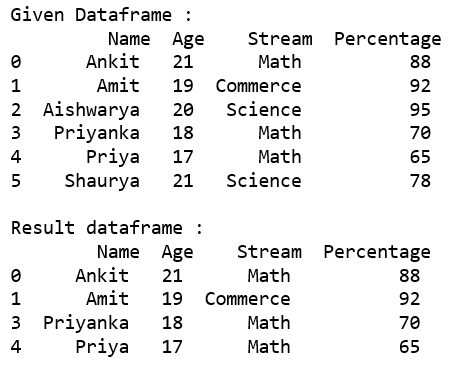Code #3 : Selecting all the rows from the given dataframe in which ‘Stream’ is not present in the options list using `.loc[]`.

 `# importing pandas ` `import` `pandas as pd ` ` `  `record ``=` `{ ` `  ``'Name'``: [``'Ankit'``, ``'Amit'``, ``'Aishwarya'``, ``'Priyanka'``, ``'Priya'``, ``'Shaurya'` `], ` `  ``'Age'``: [``21``, ``19``, ``20``, ``18``, ``17``, ``21``], ` `  ``'Stream'``: [``'Math'``, ``'Commerce'``, ``'Science'``, ``'Math'``, ``'Math'``, ``'Science'``], ` `  ``'Percentage'``: [``88``, ``92``, ``95``, ``70``, ``65``, ``78``]} ` ` `  `# create a dataframe ` `dataframe ``=` `pd.DataFrame(record, columns ``=` `[``'Name'``, ``'Age'``, ``'Stream'``, ``'Percentage'``]) ` ` `  `print``(``"Given Dataframe :\n"``, dataframe)  ` ` `  `options ``=` `[``'Math'``, ``'Science'``] ` ` `  `# selecting rows based on condition ` `rslt_df ``=` `dataframe.loc[~dataframe[``'Stream'``].isin(options)] ` ` `  `print``(``'\nresult dataframe :\n'``, rslt_df) `

Output :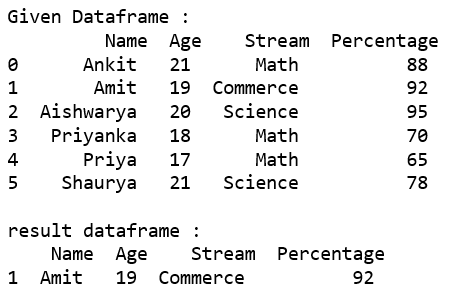### Selecting rows based on multiple column conditions using `'&'` operator.

Code #1 : Selecting all the rows from the given dataframe in which ‘Age’ is equal to 21 and ‘Stream’ is present in the options list using basic method.

 `# importing pandas ` `import` `pandas as pd ` ` `  `record ``=` `{ ` `  ``'Name'``: [``'Ankit'``, ``'Amit'``, ``'Aishwarya'``, ``'Priyanka'``, ``'Priya'``, ``'Shaurya'` `], ` `  ``'Age'``: [``21``, ``19``, ``20``, ``18``, ``17``, ``21``], ` `  ``'Stream'``: [``'Math'``, ``'Commerce'``, ``'Science'``, ``'Math'``, ``'Math'``, ``'Science'``], ` `  ``'Percentage'``: [``88``, ``92``, ``95``, ``70``, ``65``, ``78``]} ` ` `  `# create a dataframe ` `dataframe ``=` `pd.DataFrame(record, columns ``=` `[``'Name'``, ``'Age'``, ``'Stream'``, ``'Percentage'``]) ` ` `  `print``(``"Given Dataframe :\n"``, dataframe)  ` ` `  `options ``=` `[``'Math'``, ``'Science'``] ` ` `  `# selecting rows based on condition ` `rslt_df ``=` `dataframe[(dataframe[``'Age'``] ``=``=` `21``) & ` `          ``dataframe[``'Stream'``].isin(options)] ` ` `  `print``(``'\nResult dataframe :\n'``, rslt_df) `

Output :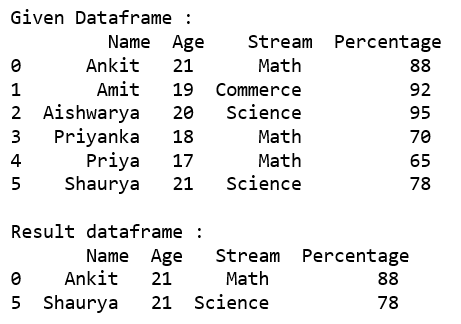Code #2 : Selecting all the rows from the given dataframe in which ‘Age’ is equal to 21 and ‘Stream’ is present in the options list using .loc[].

 `# importing pandas ` `import` `pandas as pd ` ` `  `record ``=` `{ ` `  ``'Name'``: [``'Ankit'``, ``'Amit'``, ``'Aishwarya'``, ``'Priyanka'``, ``'Priya'``, ``'Shaurya'` `], ` `  ``'Age'``: [``21``, ``19``, ``20``, ``18``, ``17``, ``21``], ` `  ``'Stream'``: [``'Math'``, ``'Commerce'``, ``'Science'``, ``'Math'``, ``'Math'``, ``'Science'``], ` `  ``'Percentage'``: [``88``, ``92``, ``95``, ``70``, ``65``, ``78``]} ` ` `  `# create a dataframe ` `dataframe ``=` `pd.DataFrame(record, columns ``=` `[``'Name'``, ``'Age'``, ``'Stream'``, ``'Percentage'``]) ` ` `  `print``(``"Given Dataframe :\n"``, dataframe)  ` ` `  `options ``=` `[``'Math'``, ``'Science'``] ` ` `  `# selecting rows based on condition ` `rslt_df ``=` `dataframe.loc[(dataframe[``'Age'``] ``=``=` `21``) & ` `              ``dataframe[``'Stream'``].isin(options)] ` ` `  `print``(``'\nResult dataframe :\n'``, rslt_df) `

Output :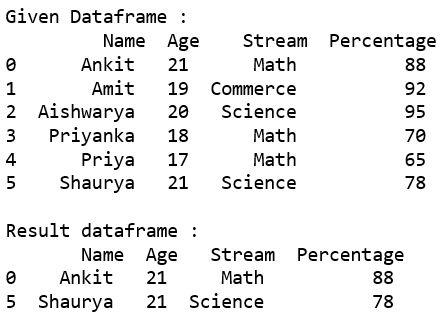My Personal Notes arrow_drop_up
Related Articles